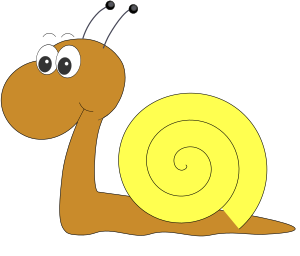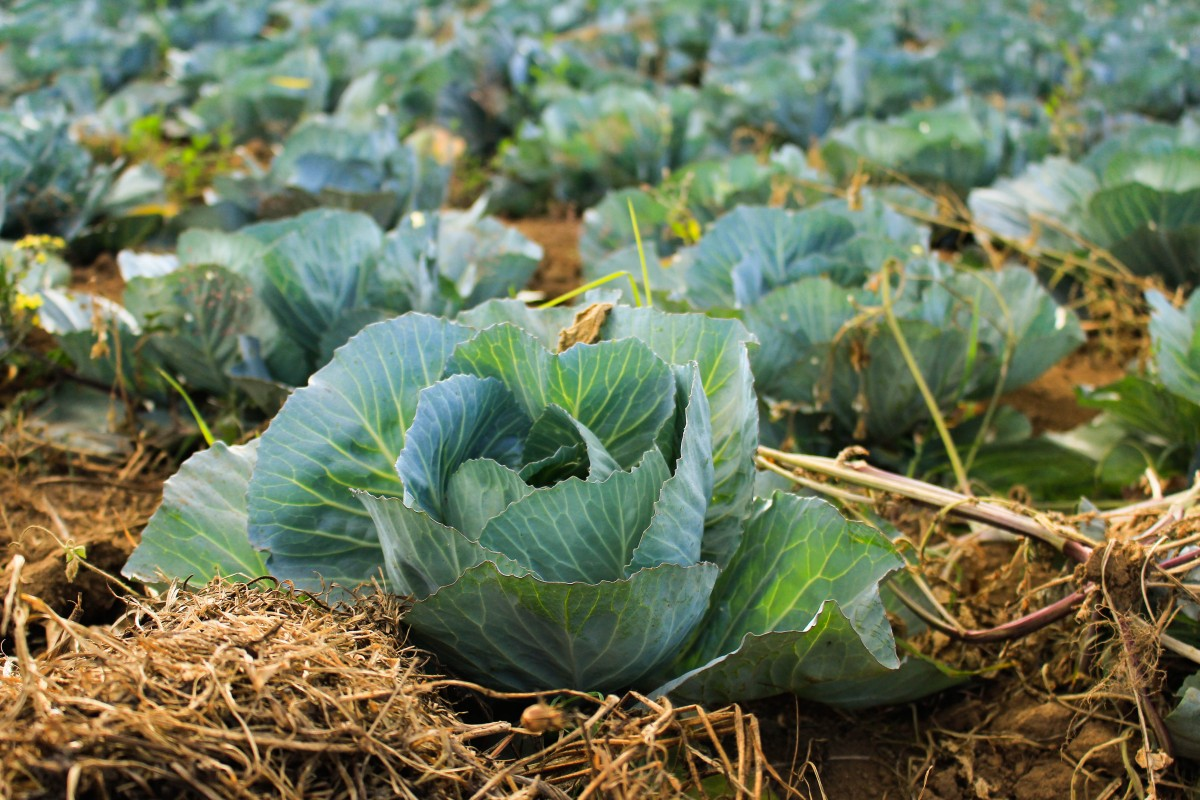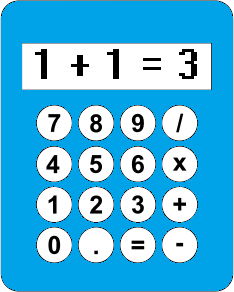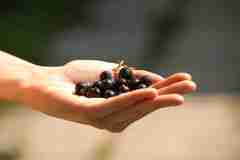# Introduction to Estimation

Estimation is a very important skill. It isn't just important in mathematics. It can come in handy in our day to day livesHave you ever entered a jelly bean guessing competition? If you haven't, the idea is to guess the number of jelly beans in a jar. Each person writes down their guess, and the person with the guess that's closest to the number of jelly beans in the jar wins the jar of jelly beans. If you could get very good at estimating things, you would have a real advantage in a jelly bean guessing competition!

• if a bill is correct.
• how far something is away, how big something is, how heavy something is or the angle between things.
• how much your grocery bill is likely to be.

If you learn to estimate, you might be able to get a good idea of how many flowers will fit in a flower bed, how many people will fit in a room, or how many people are at a cricket match.

When we do mathematics, we often worry about getting the answer exactly right. However, when we want to check whether an answer is possible, or we want to work out how far something is away, it doesn't really matter if the estimate we make isn't quite right. It really doesn't make much difference if something is 13.121 kilometres away or 13 kilometres away. An approximate answer is good enough.

Estimation gives you a quick answer that is near enough to the correct answer. It does not give the exact answer that you can sometimes get by doing calculations.

## Estimation and Bills

Sam, Al and I went to a restaurant the other day. We ordered three dishes that were $\21.99$ each and three drinks that were $2.99$ each. When we received our bill, it came to $\93.47$.

This seemed a bit high to me, so I used estimation to check. The dishes were about $\22$ each, so three dishes should have been about $\66$, and the drinks were about $\3$ each, so they should have come to about $\9$. Our total bill should have been about $\75$ dollars. It was quite a bit more than that, so I asked to have it checked. When it was rechecked, the bill came to $\74.94$, almost $\ 20$ less than the original bill. This was pretty close to my estimate. Being able to estimate saved me quite a bit of money!

## Saving Time Using EstimationGus the snail is working on his garden again. He has decided to plant a new cabbage garden that measures 63 centimetres by 49 centimetres. He is at the garden centre, hoping to buy some new punnets of cabbage plants. Each punnet contains $10$ plants, and cabbages have to be planted $12$ centimetres away from other cabbages. Gus needs to work out how many punnets of cabbages to buy.

We can use estimates to help Gus out. Each row is about $60$ centimetres long, so Gus will be able to plant about $5$ cabbages in each row ($12 \times 5 = 60$). The garden is about $48 = 4 \times 12$ centimetres wide, so Gus can plant $4$ rows of cabbages. All together, Gus can plant about $5 \times 4 = 20$ cabbages, so he needs to buy $2$ punnets of cabbages.You might be thinking that you have a calculator or computer, so you don't need to worry about learning to estimate. But the fact is, you still do! Sometimes you might press the wrong buttons on your calculator, or the calculator or computer might simply make a mistake because of a programming error. Learning to estimate will help you to catch those errors quickly. You'll know what sort of answer to expect, and will be able to catch it quickly when something is wrong.

For example, today I used the calculator on my phone to add up the numbers $1,2,3,4,5,6$ and $7$. It gave me the answer of $88$. I knew, immediately, (because I was able to estimate) that this answer was wrong. The correct answer is actually $28$, which is quite a bit less than $88$. It's always a good idea to use your brain to double check everything.

## Estimating Things Other Than the Results of CalculationsWe can use estimation for things other than the results of calculations. For example, the picture shows someone holding some grapes in their hand to estimate their weight. You might use your thumb, your hand or paces to estimate the length of something. Scouts learn to use sticks to estimate how far something is away from them. There are lots of different estimation techniques that can help you out in different situations.

## How do I get good at estimating things?

I'm glad you asked! Like any skill, the only way to get good at estimation is to do lots of practice:

• Every time you do a calculation, check whether your answer is possible by doing estimations.
• When you go to the supermarket, try to estimate the bill by adding up estimates for each of the prices. Compare your answer to the total bill to see how good your estimate was.
• Try and estimate the time it might take you to do things, like read a chapter of a book, get home from school or do your maths homework.

### Description

This mini book covers the core of Math for Foundation, Grade 1 and Grade 2 mathematics including

1. Numbers
3. Subtraction
4. Division
5. Algebra
6. Geometry
7. Data
8. Estimation
9. Probability/Chance
10. Measurement
11. Time
12. Money
13. and much more

This material is provided free of cost for Parent looking for some tricks for their Prekinder, Kinder, Prep, Year 1 and Year 2 children

### Learning Objectives

These lessons are for kids aged 4-8 with the core objective to expose their brains to concepts of addition, subtraction, division, algebra and much more.

Author: Subject Coach
You must be logged in as Student to ask a Question.